person
Open a College

Computer Science Arts English Psychology Yoga Entrepreneurship
but you can still pick the better path.
AstraPath is a growing collection of free resources you can use to learn a new subject or skill, ranging from video lectures to text-based tutorials.
by coco Drawabox by guest by Starswheat by Starswheat IB Guides by astra Problem Set 1 Solutions by elizabethmcclaire by 270392840
and learn from others.
AstraNote not just your notepad, but also a web of notes. Simply put, you get to use notes from other learners, and others can also see yours.
Of course, you also have the option to keep all your notes private.

123

## 2.1.1 Define displacement, velocity, speed and acceleration.

Displacement
Displacement is the distance moved in a particular direction. It is a vector quantity.

SI unit: m
Symbol: s

Velocity
Velocity is the rate of change of displacement. It is a vector quantity.
Velocity = (change in displacement / change in time)

SI unit: m s-1
Symbol: v or u

Speed
Speed is the rate of change of distance. It is a scalar quantity.
Speed = (change in distance / change in time)

SI unit: m s-1
Symbol: v or u

Note that speed and velocity are not the same thing. Velocity has a direction.

Acceleration
Acceleration is the rate of change of velocity. It is a vector quantity.
Acceleration = (change in velocity / change in time)

SI unit: m s-2
Symbol: a

Note that acceleration is any change in velocity, meaning an increase or decrease in velocity or a change in direction.

## 2.1.2 Explain the difference between instantaneous and average values of speed, velocity and acceleration.

Instantaneous
An instantaneous value of speed, velocity or acceleration is one that is at a particular point in time.

Average
An average value of speed, velocity or acceleration is one that is taken over a period of time.

## 2.1.3 Outline the conditions under which the equations for uniformly accelerated motion may be applied.

The equations of uniformly accelerated motion can only be under conditions where the acceleration is constant.

The equations of uniformly accelerated motion are as follows:

 Variable Symbol t time taken s distance travelled u initial velocity v final velocity a acceleration

Table 1.2.1 - Variables used in uniformly accelerated motion equations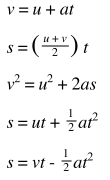Other equations may be derived from these equations.

## 2.1.4 Identify the acceleration of a body falling in a vacuum near the Earth?s surface with the acceleration g of free fall.

When we ignore the effect of air resistance on an object falling down to earth due to gravity we say the object is in free fall. Free fall is an example of uniformly accelerated motion as the only force acting on the object is that of gravity.

On the earths surface, the acceleration of an object in free fall is about 9.81 ms-1. We can easily recognise the uniform acceleration in displacement - time, velocity - time and acceleration - time graphs as shown below:

## 2.1.5 Solve problems involving the equations of uniformly accelerated motion.

A car accelerates with uniformly from rest. After 10s it has travelled 200 m.

Calculate:

Its average acceleration

S = ut + 1/2 at²

200 = 0 x 10 +  1/2  x a x 10²

200 = 50a

a = 4 m s-2

Its instantaneous speed after 10s

v² = u ² + 2as

= 0 + 2 x 4 x 10

= 80

V= 8.9 m s-1

## 2.1.6 Describe the effects of air resistance on falling objects.

Air resistance eventually affects all objects that are in motion. Due to the effect of air resistance objects can reach terminal velocity. This is a point by which the velocity remains constant and acceleration is zero.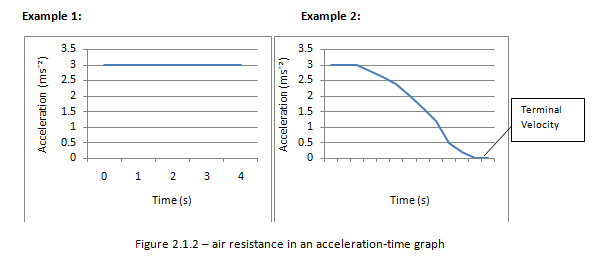In the absence of air resistance all objects have the same acceleration irrespective of its mass.

## 2.1.7 Draw and analyse distance?time graphs, displacement?time graphs, velocity?time graphs and acceleration?time graphs.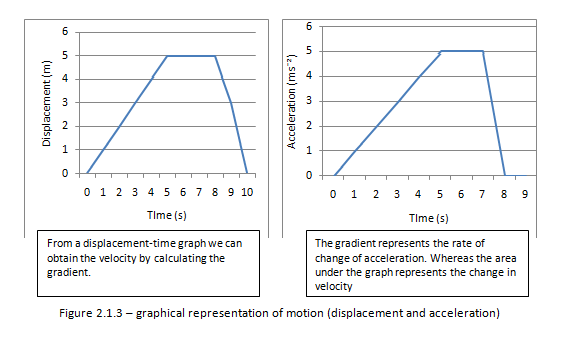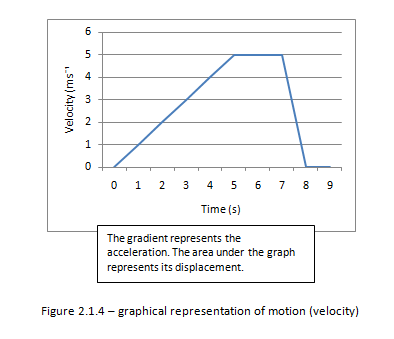## 2.1.8 Calculate and interpret the gradients of displacement?time graphs and velocity?time graphs, and the areas under velocity?time graphs and acceleration?time graphs.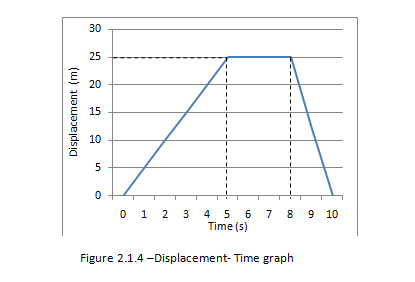Determining its velocity
We know that the gradient of a displacement – time graph gives us its velocity. Therefore for the first 5 seconds the speed is:

25/5 =5ms?¹

After the first 5 s the object is stationary for 3 s. For these 3s its velocity is zero.

After 8s the object starts to return at a faster speed then before. From the graph we find the speed to be:

25/2 =12.5ms?¹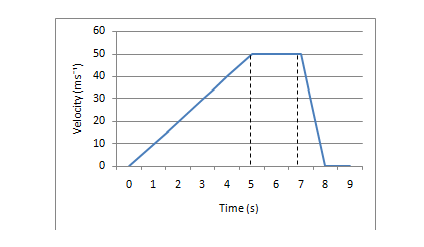Figure 2.1.5 – Velocity -Time graph

Determine its acceleration
We know that the gradient of a velocity- Time graph gives us its acceleration. Therefore for the first 5 s the acceleration is:

50/5 =10 ms?²

When the object is at constant speed from 5s to 7s its acceleration is zero. During the last second of the objects journey the object is decelerating at:

50/1 =50 ms?²

Determine its displacement
The area under a velocity-time graph is the displacement. During the first 5 s the object has travelled:

½ x 5 x 50 = 125m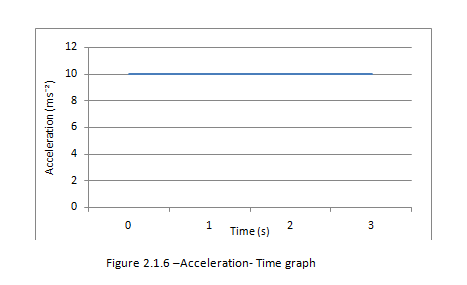Determine the change in velocity
The area under the acceleration- Time graph gives us the change in velocity

From the graph we find that the change in velocity is 10 x 3 = 30 ms?¹

Note: The gradient of the acceleration - time graph is actually the rate of change of acceleration. However it isn’t often useful.

## 2.1.9 Determine relative velocity in one and in two dimensions.

#### Problem Set 1 Solutions

```#1a:
annual_salary = float(input('Enter your annual salary: '))
portion_saved = float(input('Enter the percent of your salary to save, as a decimal:​ '))
total_cost = float(input('Enter the cost of your dream home: '))
current_savings = 0
portion_down_payment = 0.25
down_cost = total_cost * portion_down_payment
r = 0.04
monthly_salary = annual_salary/12
num_months = 0

while current_savings < down_cost:
current_savings += monthly_salary * portion_saved + current_savings * r/12
num_months += 1

num_years = num_months/12

print('Number of months:', num_months)
print('Number of years:',num_years)

#1b
annual_salary = float(input('Enter your annual salary: '))
portion_saved = float(input('Enter the percent of your salary to save, as a decimal:​ '))
total_cost = float(input('Enter the cost of your dream home: '))
semi_annual_raise = float(input('Enter the semi­annual raise, as a decimal: '))
current_savings = 0
portion_down_payment = 0.25
down_cost = total_cost * portion_down_payment
r = 0.04
monthly_salary = annual_salary/12
num_months = 0

while current_savings < down_cost:
current_savings += monthly_salary * portion_saved + current_savings * r/12
num_months += 1
if num_months%6 == 0:
monthly_salary *= (1 + semi_annual_raise)

num_years = num_months/12

print('Number of months:', num_months)
print('Number of years:',num_years)

#1c
def whatrate():
annual_salary = int(input('Enter the starting salary: '))
total_cost = 1000000
semi_annual_raise = 0.07
current_savings = 0
portion_down_payment = 0.25
down_cost = total_cost * portion_down_payment
r = 0.04
monthly_salary = annual_salary/12
num_months = 36
high = 10000
low = 0
ans = (high + low)/2
numofsteps = 0

max_savings = 0
for i in range(1,num_months+1):
max_savings += monthly_salary + current_savings * r/12
if i%6 == 0:
monthly_salary *= (1 + semi_annual_raise)
if max_savings < down_cost:
return print('It is not possible to pay the down payment in three years.')

min_savings = 0
for i in range(1,num_months+1):
min_savings += monthly_salary * 0.0001 + current_savings * r/12
if i%6 == 0:
monthly_salary *= (1 + semi_annual_raise)
if min_savings > down_cost:
return print('You don\'t really need to save, mate.')

while abs(current_savings - down_cost) > 100:
current_savings = 0
monthly_salary = annual_salary/12
numofsteps += 1
for i in range(1,num_months+1):
current_savings += monthly_salary * ans/10000 + current_savings * r/12
if i%6 == 0:
monthly_salary *= (1 + semi_annual_raise)
if current_savings > down_cost:
high = ans
else:
low = ans
ans = (high + low)/2
print('Best savings rate:', round(ans/10000,4))
print('Steps in bisection search:​', numofsteps)```

#### MIT 6.0001

# Lecture 1: What is Computation?
1.1 Topics of the courses
* iteration and recursion
* organize and modularize
* ALGORITHMS!
* complexity of algorithms
1.2 Construction of languages
* primitive constructs - numbers, strings, simple operators
* syntax - 3.2 * 5
* static semantics - syntactically valid strings have meaning; e.g. 3.2 * "hi" #static semantic error
* semantics - meaning associated with a syntactically correct string of symbols with no static semantic errors
# syntactic + static semantic + semantic = all those errors come from
1.3 Objects (or type)
1.3.1 scalar object (can not be subdivided)
* int - represents integers, ex. 5
* float - represent real numbers, ex. 3.27
* bool - represent Boolean values True and False
* Nonetype - special and has one value, None
# type() - see the type of an object
# int() or float() - type conversions (cast)
1.3.2 non-scalar (internal structure)
* list - [1, 2, 3]
1.4 Expression <object><operator><object>
# an expression has a value, which has a type
* i + j - the sum
* i - j - the difference
* i * j - the product
* i / j - the division #result is float
** i % j - the remainder when i is divided by j
** i ** j - i to the power of j
# use parentheses () to tell Python to do these operations first
1.5 Binding variables and values
* equal sign is an assignment of a value to a variable name
* pi = 3.14159; radius = 2.2; area = pi * (radius ** 2)

# Lecture 2: Branching and iteration
2.1 string object type
2.1.1 strings - letters, special characters, spaces, digits
* hi = "hello there"; name = "qixiang"; - enclose in quotation marks or single quotes
* greeting = hi + " " + name - concatenate strings
* silly = hi + " " + name * 3 - operations on string
2.1.2 INPUT/OUTPUT - print("") & input("")
* 2 way of printing sentences - print("My name is", x, "."); print("My name is " + x + " .")
2.1.3 comparision operators on int, float, string (ascii table)
* i == j - equality test
* i != j - inequality test
2.1.4 logic operators on bools
* not a - True if a is False, False if a is True
* a and b - True if both are True
* a or b - True if either or both are True
2.2 branching and conditionals (CONTROL FLOW)
2.2.1 if<condition> + <expression>
2.2.2 elif<condition> + <expression>
2.2.3 else: +<expression>
* <condition> has a value True or False
* evaluate expressions in the block if <condition> is True
2.3 indentation - how you denote (indiate) block of code
* vs == and !=
2.4 iteration and loops
2.4.1 while loops
* while <condition>: <expression>
# <condition> evaluates to a Boolean;
if <condition> is True do <expression>;
check <condition> again;
repeat until <condition> is False
* iteration through nums in a sequence - i = 0; while num < i: <expression>
2.4.2 for loops
* for <variable> in range(start, stop, step)
# loop until value is stop -1
2.4.3 break STATEMENT
* exits only innermost loop!
2.4.4 comparation between for and while LOOPS
* for LOOPS -
# known number of iterations;
with an inner counter;
rewritiable through while LOOPS
* while LOOPS -
# unbounded number of iterations;
can use a counter but must initialize;
MAYNOT rewritiable through for LOOPS

# Lecture 3: String Manipulation, Guess and Check, Approximations, Bisection
# last 3 are ALGORITHMS for guessing
3.1 string manipulation
3.1.1 definition and basic operation
* sequence of case sensitive characters
* compare operation - ==, >, < etc.
* length operation - len()
3.1.2 indexing
* square brackets to index string
# s = "abc" index: 0 1 2 or -3 -2 -1
s == s[-3] = "a"; s == s[-2] = "b"; s == s[-1] = "c"
3.1.3 slicing
* slice strings using [start: stop: step]
# s = "abcdefgh"
s[3: 6: 2] = "df"
* strings are "IMMUTABLE" - cannot be modified
# change s = "hello" to s = "yello"
s = 'y' - ERROR!
s = 'y' + s[1: len(s)]
3.1.4 LOOPS RECAP (string loops)
* loop variable
- numbers - for var in range(4, 6): <expression>
- indexing in string - for index in range(len(s)): <expression>
- CHAR - for char in s: <expression>
# more pythonic for char in word
* example - Robot cheerleader & Common letter
3.2 guess and check algorithms (exhuasted enumeration)
* for guess in range(cube + 1) - test from 0 to cube
if guess ** 3 == cube:
print("Cube root of", cube, "is", guess)
# guess a value for solution
# check if the solution is correct
# keep guessing and checking ...unitil find solution or guessed all values
3.3 approximate solutions (find an approximate solution)
* Initial value
- epsilon (how close to the target) and increment (how large each step is)
- target and guess, and num_guesses
* while abs(guess ** 3 - cube) >= epsilon and guess <=cube:
guess += increment
num_guesses += 1
# good enough approximation
# keep guessing if abs(guess ** 3 - cube) >= epsilon, for some small epsilon
# decreasing increment size - slower program
increasing epsilon - less accurate answer
3.4 bisection search method
* half interval each iteration and new guess is halfway in between
* guess CONVERGES on the order of log2N steps
* Initial low and high value - low = 0, high = guess, guess = (high + low) / 2.0
While abs(guess ** 3 - cube) >= epsilon:
if guess ** 3 < cube:
low = guess  #re-define the low boundary
else:
high = guess  #re-define the high boundary
guess = (high + low) / 2.0
num_guesses +=1

#### Journal - 6 Oct 2020

It was a wonderful day.

#### Introduction to Computational Thinking and Data Science

Lecture 1

1. Computational models: optimisation, simulation, statistical

2. Lambda function

3. Optimisation model: optimise with constraints

a. Brute force algorithm

b. Greedy algorithm (doesn't always yield the best result)

Lecture 2

Brute force algorithm

1. Left-most and depth-most enumeration

Paused at 13:00

#### Startup Lessons

Lecture 1.

• Be real builders. Real recognise real.
• Too much money, plenty of good people, and plenty of good problems to solve.

Lecture 2.

• Show, don't tell
• Don't use buzzwords
• Write using journalism's inverted pyramid

#### Introduction

Lecture 1

Concepts

• computer - calculations, storage, result memorization, - tell them what to do!
• 1. statement of facts  2. order/recipe/ the sequence of steps
• flow of control: making decisions
• 6 primitives --> types of result -- > instruction flow --> output
• expression: primitives + programming languages
• expression - value - type

[Revisit]

Programming

• 35:00
• give names, reuse names
• = assignment (left - variable)

Remaining Question:

#### `u``sa_gold = 46``uk_gold = 27``romania_gold = 1``total_gold = usa_gold + uk_gold + romania_gold``print(total_gold)``romania_gold += 1`

```    total_gold = usa_gold + uk_gold + romania_gold
print(total_gold)```

My question:

Why do we get 75 for the second print?

From John's notes:

so, romania_gold + = 1 is equal to romania_gold = romania gold + 1

#### Personality and its Transformations

Lecture 1

1. Spans a wide range of disciplines

2. We've been shaping how all of us behave as long as we've been social

3. Mythological representation and shamanic transformation

4. Piaget

5. Jung

6. Freud

Lecture 2

1. Depth psychologist

2. Constructivist

3. Humanist

4. Existentialist

5. Phenomenologist

Lecture 3

1. Archetypal stories: we somehow know what makes a story interesting

2. Constituent elements of stories: characters

#### Introduction to Psychology

Lecture 1:
With Mind Palace, memorizing a whole book can be both fun and easy.
Mind Palace is a memory technique invented by Greek poet, Simonides of Ceos, beloved by Sherlock Holmes, and used by memory champions.
Create a web app using Django Drawing for charity

This feature is still in beta. Leave your email to
get early access.

Learn by doing real projects provided big companies, startups, and NGOs.
If you don't practise, you're not really learning.
If you don't know how to practise, you won't pick up a skill.
Start a company Get a job at Tesla

This feature is still in beta. Leave your email to
get early access.

Launch your career while you're learning.
With AstraConnect, you can connect with companies and organisations early and learn on-demand skills.
Or, you can connect with startup founders and investors to launch your startup--that's how Astrasum was born!

#### Sunday 25 Oct 2020

Learn computer science on Astrasum ...

### Routines:

- Learn computer science on Astrasum
- Every Sunday
...
Mastery comes from the power of repetition
Set your routines on AstraPanel and your daily to-dos will be automatically generated every morning.

## A Thousand Paths to Greatness

### Share a Path (free courses or tutorials)

×

Welcom to Astrasum

My name is Astra and I'll help you start a joyful and highly effective learning experience with Astrasum!

Before that, let's take a second to reflect a bit on how you learn, shall we?

You will probably find that in order to acquire a skill or some level of expertise on a subject, you take 5 steps that make up your learning behaviour.

1. You find the learning materials for the subject.
2. You input these materials into your brain through reading and listening.
3. You process the new information through memorising and associating in order to construct a new thinking model.
4. You practise by solving problems that are designed for learning, or by having basic conversations in the case of learning languages.
5. You apply the new skill you just acquired and start creating values for the world with it.

Sounds familiar? So what is Astrasum?

Astrasums hacks learning. Astrasum accelerates your learning by helping you become better and better at each one of these steps with our technology and growing community.

As your scroll through the home page, you'll see one window frame after another. That's AstraPath, i.e. a path that you can take to learn something new.

These are free and high-quality learning materials for a wide range of subjects handpicked by the Astrasum team and members of this community.

We encourage you to also share your own AstraPaths in this community.

If you've found an AstraPath you'd like to learn more about, click '•••' and you'll see a few options.

1. Click 'Start learning' if you want to dive into this AstraPath right away.
2. If you want to check out the original source of this AstraPath for more information, click 'Go to original source'.
3. We also encourage you to use 'Copy link' to share this AstraPath with friends who might be interested as well.

At the top, you'll find a select box 'Filter by subject', through which you can find AstraPaths in a particular subject you're interested in.

If you are already taking a course at school or elsewhere, you're welcome to create an AstraPath of you own and user other features on Astrasum to assist your learning.

Current features:

• AstraPanel - Maximise your productivity with powerful routines.
• AstraPath - Desentralised skill-oriented learning content from 20-minute crash courses to hardcore expert-level courses.
• AstraNote - Next-generation note-taking should be inter-connected--take intelligent notes in a network of a thousand different minds.
• MindPalace - Memorise with the most powerful memory technique championed by memory masters.

Upcoming features:

• AstraApprentice - Practise with real-life projects and thousands of other learners.
• AstraConnect - The whole point of learning is to be able to apply a new skill. AstraConnect helps you find a job, start a company, and explore a thousand other possibilities when you've acquired a new skill!
• AstraClassroom - Learn in the right environment in our VR classrooms
How does it work?

Scroll down and find a path (free courses or tutorials)

Click on the image and start learning!

Click '•••' to choose from other options.

## Arts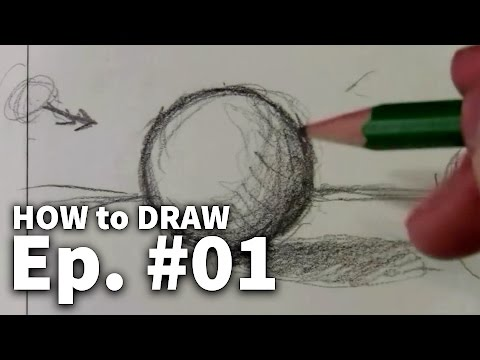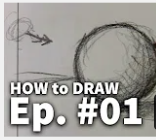Drawing for absolute beginner
more_horiz
school Subject: Arts
assessment Level: Beginner
help Prerequisite: None
assignment_turned_in Skills unlocked: Drawing with confidence
perm_media Learning media: Video lectures
`astra: "Learn to draw, sketch and more in this series of videos which explains the methods and techniques involved in learning this great skill. I demonstrate shading, lines, perspective, proportions, values, edges & more. This series starts from the very beginning to help someone who has never drawn in their life, to be able draw with confidence and have fun."`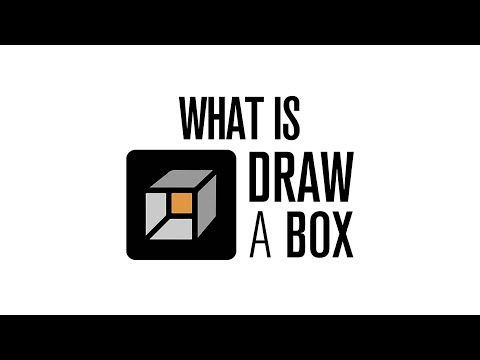Drawabox
more_horiz
school Subject: Arts
assessment Level: Beginner
help Prerequisite: None
assignment_turned_in Skills unlocked: Drawing
perm_media Learning media: Video lectures, practices
```astra: "Drawabox's goal is to provide beginners with a strong foundation, and to equip them with the things a lot of other courses and tutorials tend to take for granted."

It's a brilliant free online course for anyone interested in dabbling or starting a career in art.```